Published on

# Data types in python

Authors
•Name
Aswin Barath
@AswinBarath2

Data types are one of the building blocks of python. And You can do a lot of things with data types!

Fact: In python, all data types are implemented as an object.

A data type is like a specification of what kind of data we would like to store in memory and python has some built-in data types in these categories:

• Text type: str
• Numeric types: int, float, complex
• Sequence types: list, tuple, range
• Mapping type: dict
• Set types: set, frozenset
• Boolean type: bool
• Binary types: bytes, bytearray, memoryview

Now, let's demistify all these data types by using type() function to display the data type of the variable.

## Text type

### str

• str stands for string in python used for storing text in python.
• Strings can be written either in single quotes or double qoutes in python, hence your choice.

Example: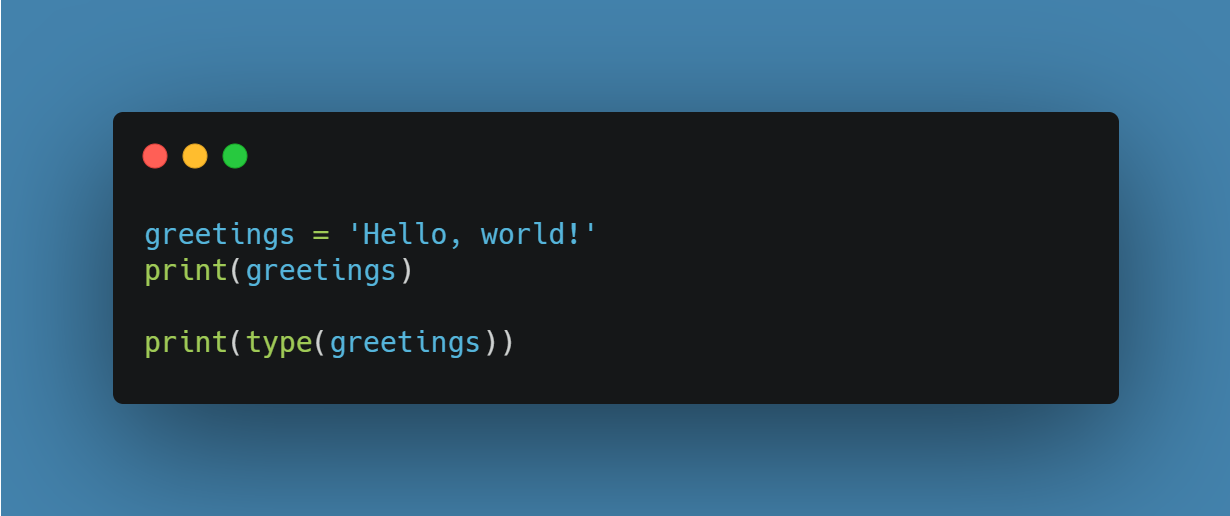Output:

``````Hello, world!
<class 'str'>
``````

## Numeric types

### int

• int stands for integer used to store integers (positive and negative numbers).

Example: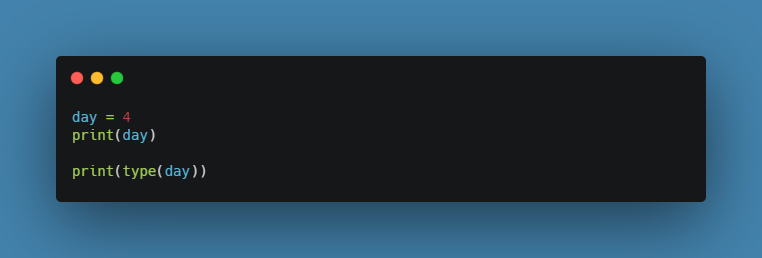Output:

``````4
<class 'int'>
``````

### float

• float stands for floating-point numbers (decimal point numbers)

Example:Output:

``````3.14
<class 'float'>
``````

### complex

• Complex numbers have a real and imaginary part, which are each a floating point number.
• Complex numbers can be written in two forms:
1. real + (imag)j
2. complex(real, imag)

Example: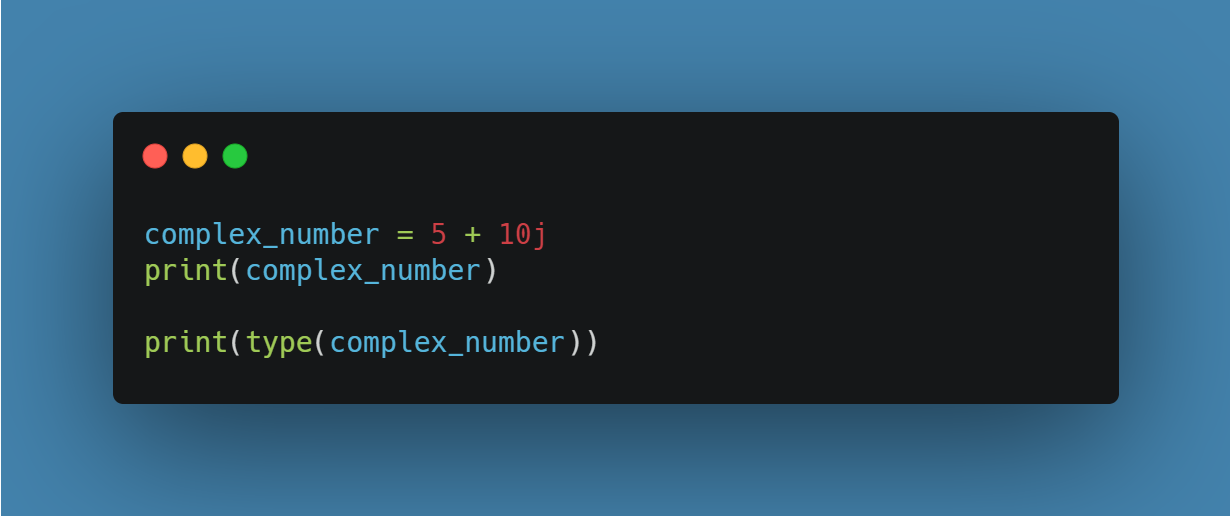Output:

``````(5+10j)
<class 'complex'>
``````

## Sequence types

### list

• A list is data type where you can store a collection of data
• A list can also contain different data types
• A list is ordered and changeable and allows duplicate members

Example: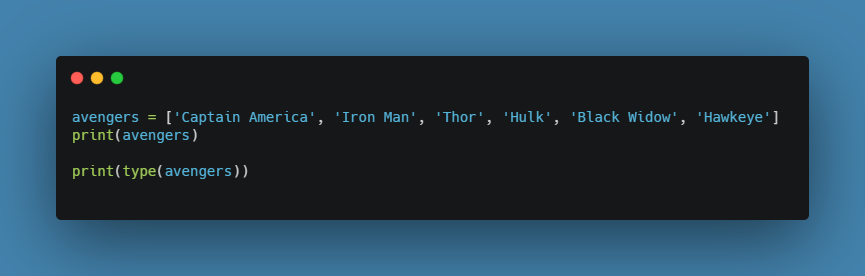Output:

``````['Captain America', 'Iron Man', 'Thor', 'Hulk', 'Black Widow', 'Hawkeye']
<class 'list'>
``````

### tuple

• A tuple is data type where you can store a collection of data
• A tuple can also contain different data types
• A tuple is ordered and unchangeable and allows duplicate members

Example:Output:

``````('Captain America', 'Iron Man', 'Thor', 'Hulk', 'Black Widow', 'Hawkeye')
<class 'tuple'>
``````

### range

• The range type represents an immutable (unchangable) sequence of numbers
• Commonly used for looping a specific number of times in for loops.

Example: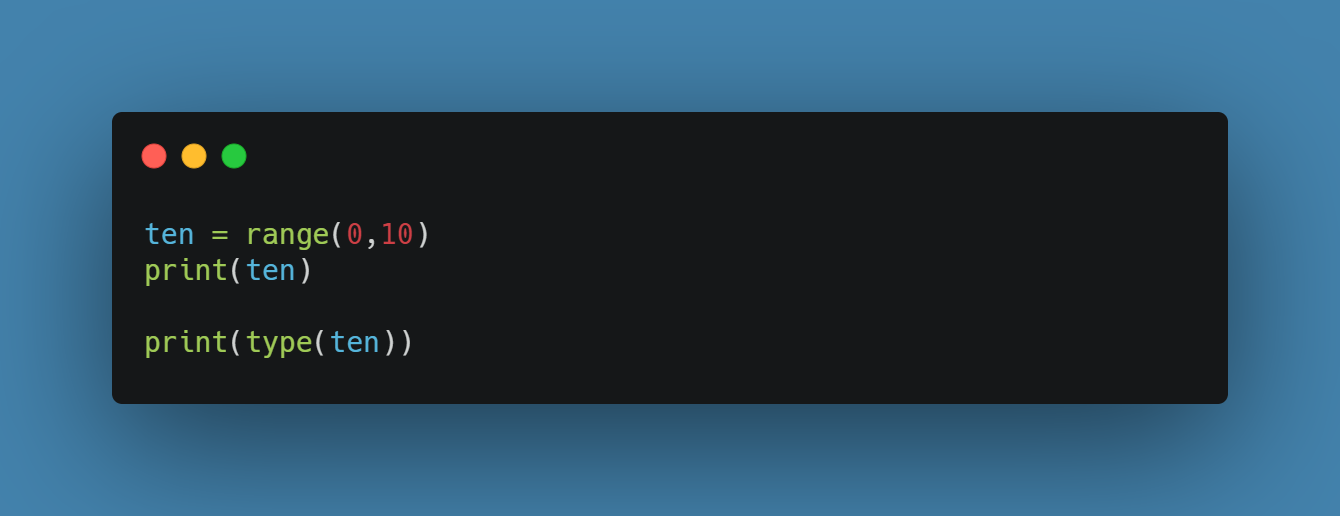Output:

``````range(0, 10)
<class 'range'>
``````

## Mapping type

### dict

• dict stands for dictionary in python
• Dictionaries are used to store data values in key:value pairs
• A dictionary is a collection which is unordered, changeable and does not allow duplicates

Example: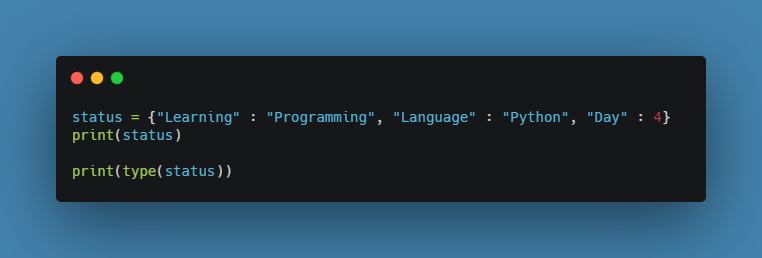Output:

``````{'Learning': 'Programming', 'Language': 'Python', 'Day': 4}
<class 'dict'>
``````

## Set types

### set

• A set is data type where you can store a collection of data
• A set can also contain different data types
• A set is unordered and unindexed and allows no duplicate members

Example: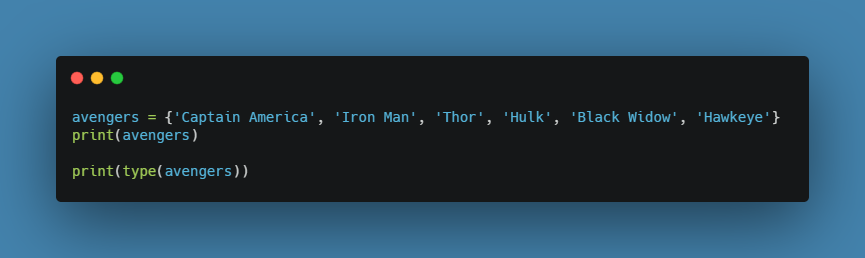Output:

``````{'Black Widow', 'Iron Man', 'Thor', 'Hawkeye', 'Hulk', 'Captain America'}
<class 'set'>
``````

### frozenset

• frozenset data type can be created by frozenset() function
• The frozenset() function accepts an iterable and returns an unchangeable frozenset object (which is like a set object, only unchangeable)

Example: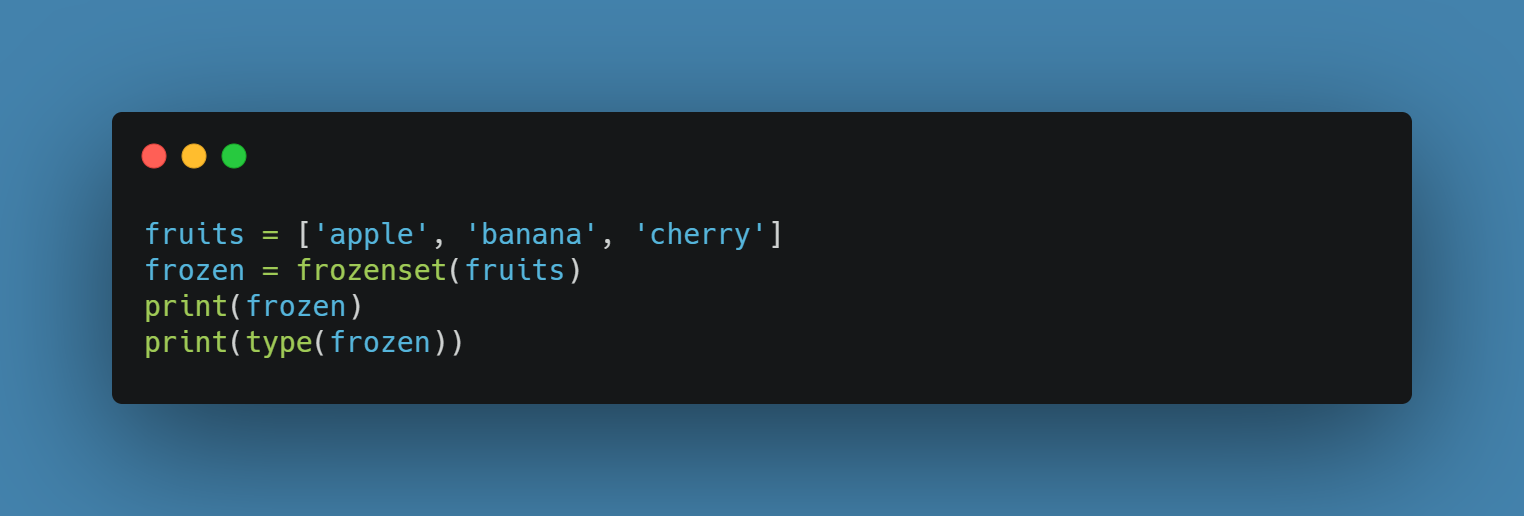Output:

``````frozenset({'cherry', 'banana', 'apple'})
<class 'frozenset'>
``````

## Boolean type

### bool

• bool stands for boolean in python
• Booleans represent one of two values: True or False

Example: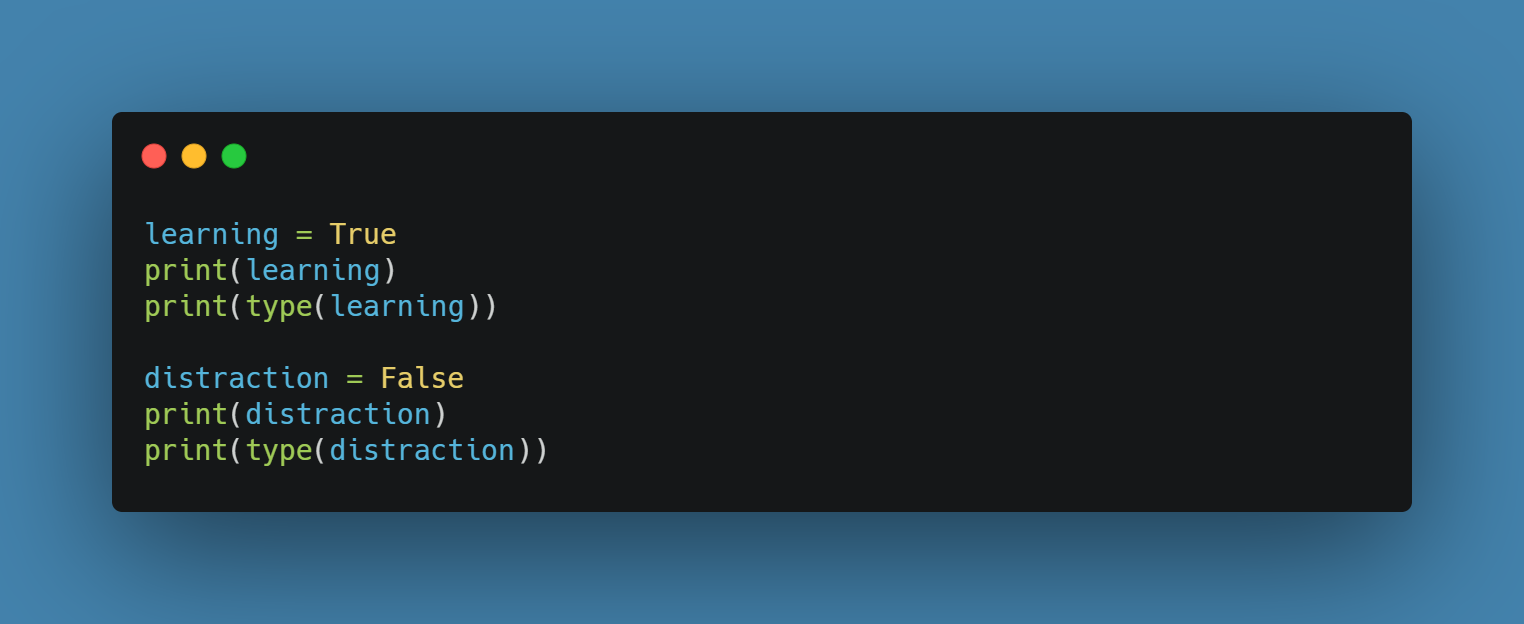Output:

``````True
<class 'bool'>
False
<class 'bool'>
``````

## Binary types

### bytes

• bytes data type can be created in two forms:
1. bytes() function
2. prefix 'b'

Example: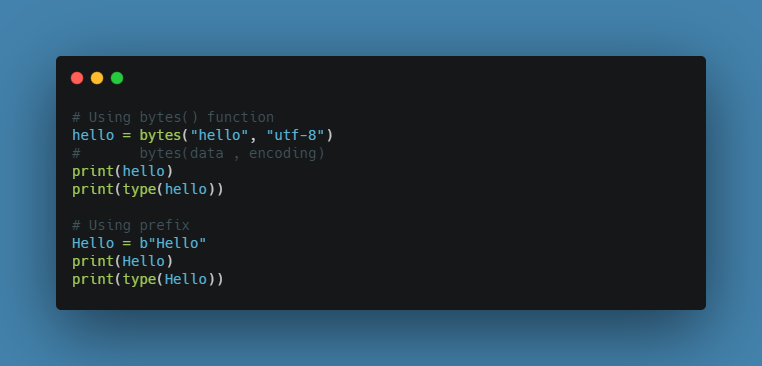Output:

``````b'hello'
<class 'bytes'>
b'Hello'
<class 'bytes'>
``````

### bytearray

• bytearray() function returns a bytearray object
• It can convert objects into bytearray objects

Example: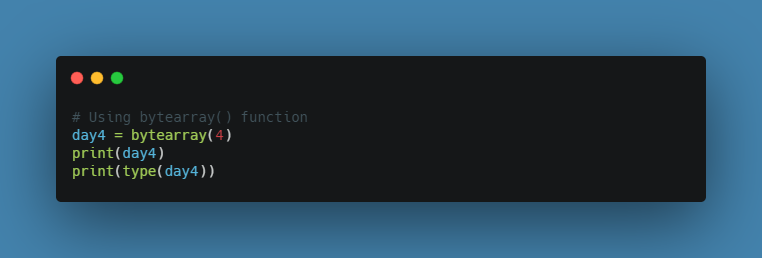Output:

``````bytearray(b'\x00\x00\x00\x00')
<class 'bytearray'>
``````

### memoryview

• memoryview() function returns a memory view object from a specified object

Example: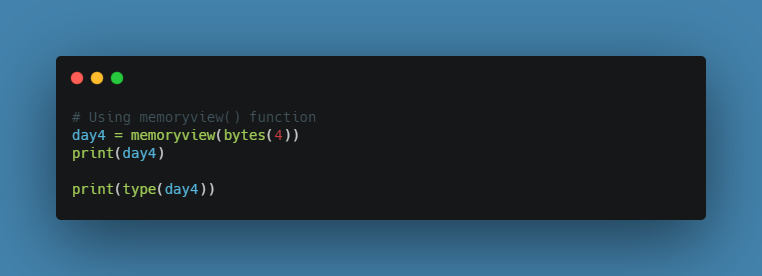Output:

``````<memory at 0x2b4f7a8a7408>
<class 'memoryview'>
``````

#### Note

As you might have observed earlier, some data types can be also implemented using their constructors. This same technique can also be applied to every data type. Example: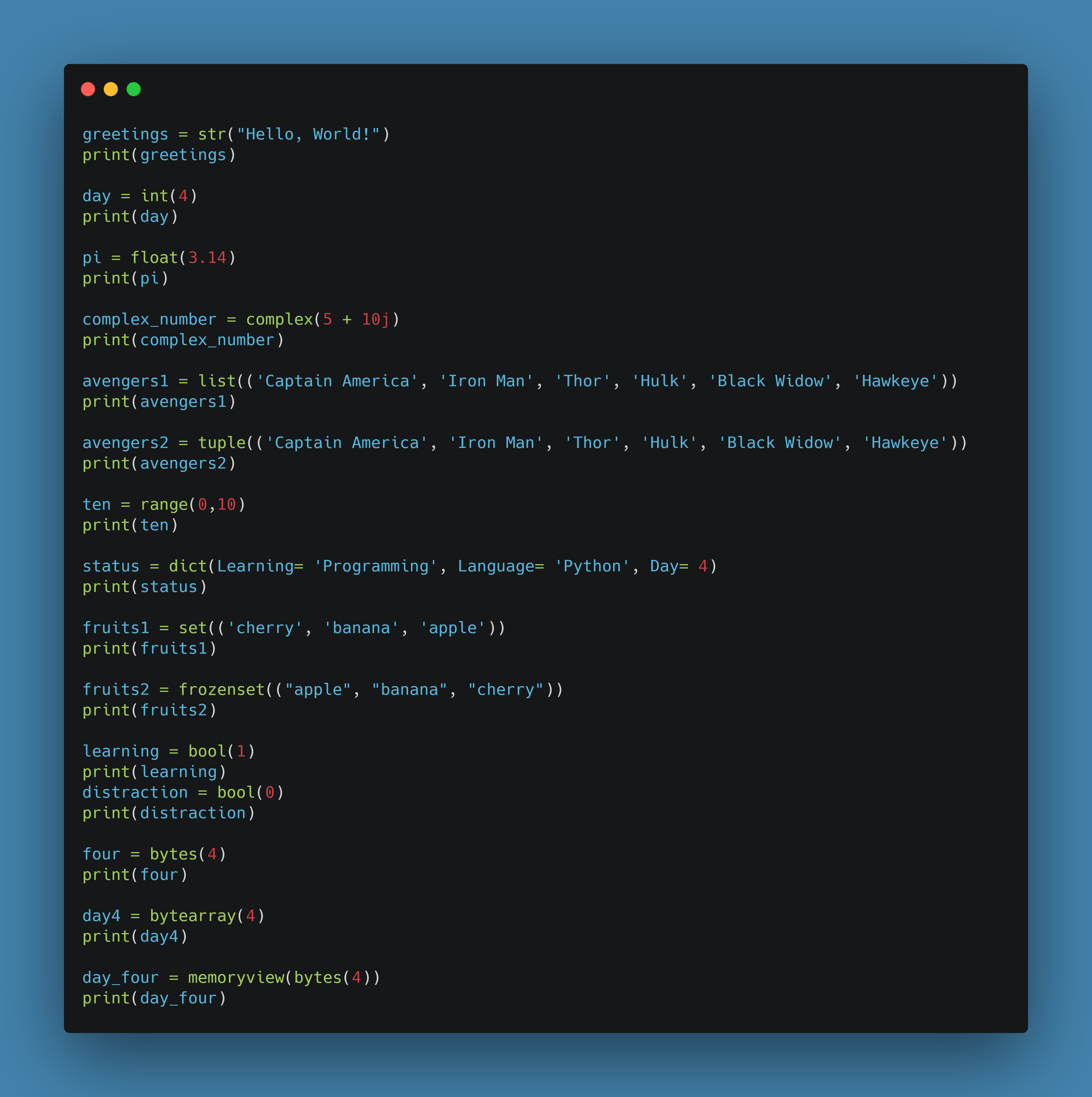Output:

``````Hello, World!
4
3.14
(5+10j)
['Captain America', 'Iron Man', 'Thor', 'Hulk', 'Black Widow', 'Hawkeye']
('Captain America', 'Iron Man', 'Thor', 'Hulk', 'Black Widow', 'Hawkeye')
range(0, 10)
{'Learning': 'Programming', 'Language': 'Python', 'Day': 4}
{'apple', 'cherry', 'banana'}
frozenset({'banana', 'cherry', 'apple'})
True
False
b'\x00\x00\x00\x00'
bytearray(b'\x00\x00\x00\x00')
<memory at 0x2b8346a29408>
``````